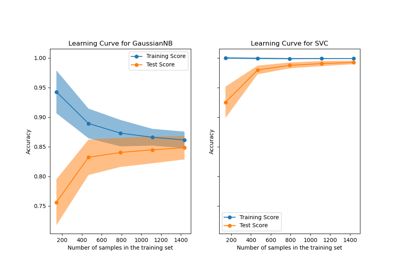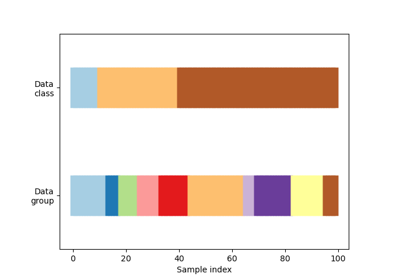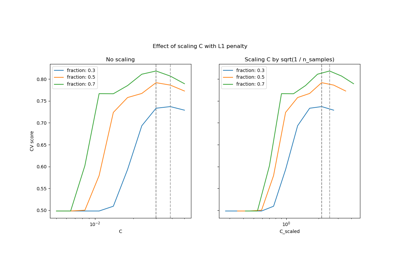# sklearn.model_selection.ShuffleSplit¶

class sklearn.model_selection.ShuffleSplit(n_splits=10, *, test_size=None, train_size=None, random_state=None)[source]

Random permutation cross-validator

Yields indices to split data into training and test sets.

Note: contrary to other cross-validation strategies, random splits do not guarantee that all folds will be different, although this is still very likely for sizeable datasets.

Read more in the User Guide.

For visualisation of cross-validation behaviour and comparison between common scikit-learn split methods refer to Visualizing cross-validation behavior in scikit-learn

Parameters:
n_splitsint, default=10

Number of re-shuffling & splitting iterations.

test_sizefloat or int, default=None

If float, should be between 0.0 and 1.0 and represent the proportion of the dataset to include in the test split. If int, represents the absolute number of test samples. If None, the value is set to the complement of the train size. If train_size is also None, it will be set to 0.1.

train_sizefloat or int, default=None

If float, should be between 0.0 and 1.0 and represent the proportion of the dataset to include in the train split. If int, represents the absolute number of train samples. If None, the value is automatically set to the complement of the test size.

random_stateint, RandomState instance or None, default=None

Controls the randomness of the training and testing indices produced. Pass an int for reproducible output across multiple function calls. See Glossary.

Examples

>>> import numpy as np
>>> from sklearn.model_selection import ShuffleSplit
>>> X = np.array([[1, 2], [3, 4], [5, 6], [7, 8], [3, 4], [5, 6]])
>>> y = np.array([1, 2, 1, 2, 1, 2])
>>> rs = ShuffleSplit(n_splits=5, test_size=.25, random_state=0)
>>> rs.get_n_splits(X)
5
>>> print(rs)
ShuffleSplit(n_splits=5, random_state=0, test_size=0.25, train_size=None)
>>> for i, (train_index, test_index) in enumerate(rs.split(X)):
...     print(f"Fold {i}:")
...     print(f"  Train: index={train_index}")
...     print(f"  Test:  index={test_index}")
Fold 0:
Train: index=[1 3 0 4]
Test:  index=[5 2]
Fold 1:
Train: index=[4 0 2 5]
Test:  index=[1 3]
Fold 2:
Train: index=[1 2 4 0]
Test:  index=[3 5]
Fold 3:
Train: index=[3 4 1 0]
Test:  index=[5 2]
Fold 4:
Train: index=[3 5 1 0]
Test:  index=[2 4]
>>> # Specify train and test size
>>> rs = ShuffleSplit(n_splits=5, train_size=0.5, test_size=.25,
...                   random_state=0)
>>> for i, (train_index, test_index) in enumerate(rs.split(X)):
...     print(f"Fold {i}:")
...     print(f"  Train: index={train_index}")
...     print(f"  Test:  index={test_index}")
Fold 0:
Train: index=[1 3 0]
Test:  index=[5 2]
Fold 1:
Train: index=[4 0 2]
Test:  index=[1 3]
Fold 2:
Train: index=[1 2 4]
Test:  index=[3 5]
Fold 3:
Train: index=[3 4 1]
Test:  index=[5 2]
Fold 4:
Train: index=[3 5 1]
Test:  index=[2 4]


Methods

 Get metadata routing of this object. get_n_splits([X, y, groups]) Returns the number of splitting iterations in the cross-validator split(X[, y, groups]) Generate indices to split data into training and test set.

Get metadata routing of this object.

Please check User Guide on how the routing mechanism works.

Returns:

A MetadataRequest encapsulating routing information.

get_n_splits(X=None, y=None, groups=None)[source]

Returns the number of splitting iterations in the cross-validator

Parameters:
Xobject

Always ignored, exists for compatibility.

yobject

Always ignored, exists for compatibility.

groupsobject

Always ignored, exists for compatibility.

Returns:
n_splitsint

Returns the number of splitting iterations in the cross-validator.

split(X, y=None, groups=None)[source]

Generate indices to split data into training and test set.

Parameters:
Xarray-like of shape (n_samples, n_features)

Training data, where n_samples is the number of samples and n_features is the number of features.

yarray-like of shape (n_samples,)

The target variable for supervised learning problems.

groupsarray-like of shape (n_samples,), default=None

Group labels for the samples used while splitting the dataset into train/test set.

Yields:
trainndarray

The training set indices for that split.

testndarray

The testing set indices for that split.

Notes

Randomized CV splitters may return different results for each call of split. You can make the results identical by setting random_state to an integer.

## Examples using sklearn.model_selection.ShuffleSplit¶Plotting Learning Curves and Checking Models’ Scalability

Plotting Learning Curves and Checking Models' ScalabilityVisualizing cross-validation behavior in scikit-learn

Visualizing cross-validation behavior in scikit-learnScaling the regularization parameter for SVCs

Scaling the regularization parameter for SVCs